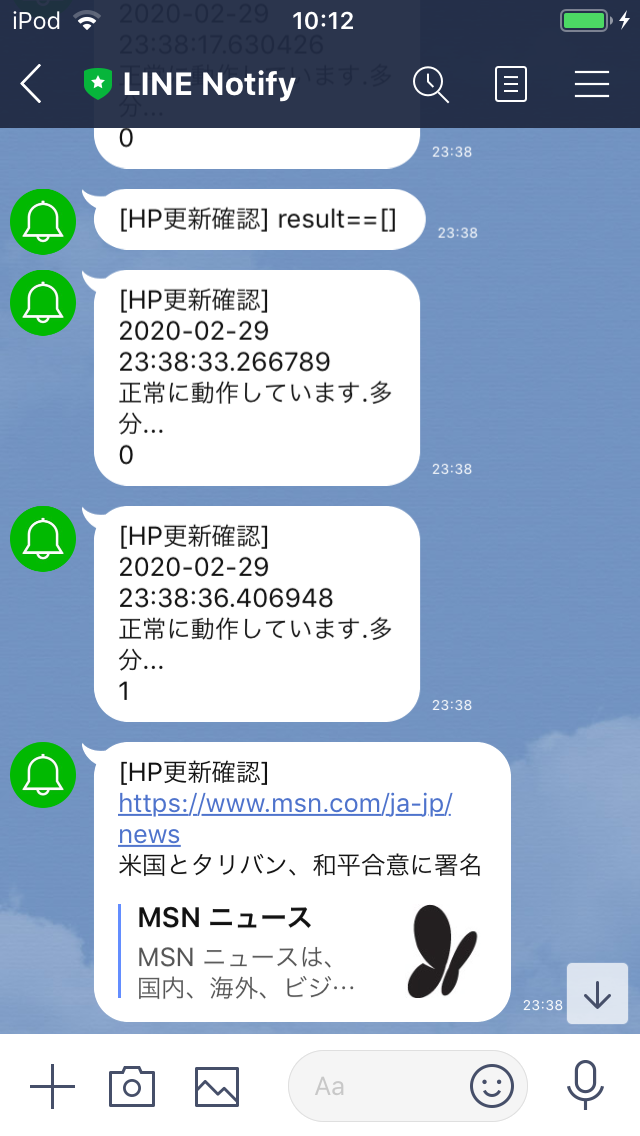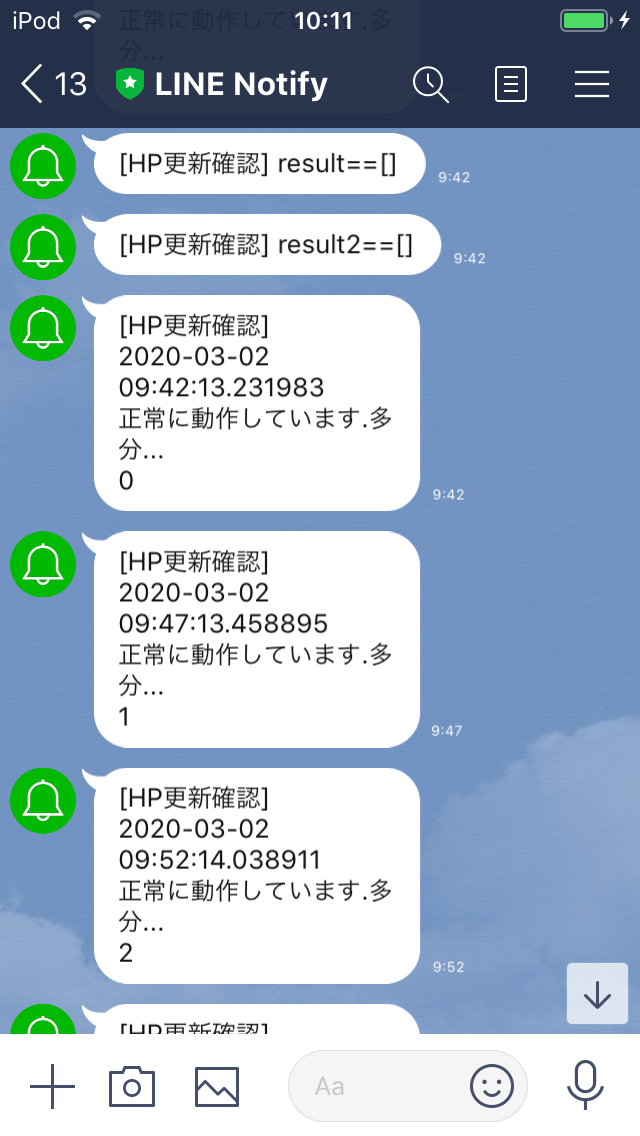# Introduction

Due to the new coronavirus infection (COVID-19), the school was closed and it would be nice to update the latest information on the school's website at any time, so I automatically got the update and made a notification system for class LINE.

# environment

``````ubuntu19.10
Python 3.7.5
``````

# Implementation method

1. Get information on the school homepage once every 30 minutes.
2. Check the difference from the previously acquired data.
3. Notify class LINE using LINE Notify if there is a difference

# program

1. Get information on the school homepage. (Get contact with the TOP page and each grade)
``````res = requests.get(url1)
res2 = requests.get(url2)
res.encoding = res.apparent_encoding
res2.encoding = res2.apparent_encoding
``````
1. Check the difference from the previously acquired data.
``````m = "\n" + link
result = list(set(list1) - set(list2))

print("raw" + str(result))

if len(result) > 30:
result = []

elif len(result) >= 30:
result = "Character limit ...(¡>Д<¡)・ ゜ ・"
result = m + "\n" + result

elif result != []:
for s in result:
m = m + "\n" + s
result = m
``````
1. Notify class LINE using LINE Notify if there is a difference
``````url = "https://notify-api.line.me/api/notify"
headers = {'Authorization': 'Bearer ' + access_token}

``````

The entire

``````import re
import requests
import copy
import time
import requests
import datetime

def get_data(url1, url2):
global res, res2
res = requests.get(url1)
res2 = requests.get(url2)
res.encoding = res.apparent_encoding
res2.encoding = res2.apparent_encoding

def format_text(text):
text = re.sub(r'[a-zA-Z0-9]', '', text)
text = re.sub(r'[!-/:[email protected][-`{-~]', '', text)
text = text.replace(' ', '')
return text

print(datetime.datetime.now())
text = format_text(res.text)
text2 = format_text(res2.text)
global l1, l2
l1 = text.split()
l2 = text2.split()
global result, result2
result = difference(l1, l_b1, URL1)
result2 = difference(l2, l_b2, URL2)

print(result, result2)

global l_b1, l_b2
l_b1 = copy.deepcopy(l1)
l_b2 = copy.deepcopy(l2)

result = list(set(list1) - set(list2))

print("raw" + str(result))

if len(result) > 30:
result = []

elif len(result) >= 30:
result = "Character limit ...(¡>Д<¡)・ ゜ ・"
result = m + "\n" + result

elif result != []:
for s in result:
m = m + "\n" + s
result = m

return result

def line(message, access_token):
url = "https://notify-api.line.me/api/notify"
headers = {'Authorization': 'Bearer ' + access_token}

def sent(name, message, token1, token2):
if message != []:
line(message, token1)
line(message, token2)
else:
line(name + "==[]", token2)

def main():
global l_b1, l_b2, URL1, URL2
l_b1 = []
l_b2 = []
URL1 = "URL of the HP you want to get"
URL2 = "URL of the HP you want to get"
class_line = "Class LINE token"

while True:
get_data(URL1, URL2)

for i in range(6):
line("\n" + str(datetime.datetime.now()) + "\n" +
"It's working fine.Perhaps..." + "\n" + str(i), admin_line)
time.sleep(300)

if __name__ == '__main__':
main()
``````

I sent myself the message "It's working fine. Maybe ..." once every 5 minutes. I'll keep it a secret that I don't really need it. (Laughs)

To be honest, the character limit is appropriate.

### Postscript

I changed main () as follows and dealt with the error.

``````
def main():
global l_b1, l_b2, URL1, URL2
l_b1 = []
l_b2 = []
URL1 = "URL of the HP you want to get"
URL2 = "URL of the HP you want to get"
class_line = "Class LINE token"

while True:
try:
get_data(URL1, URL2)

for i in range(6):
line("\n" + str(datetime.datetime.now()) + "\n" +
"It's working fine.Perhaps..." + "\n" + str(i), admin_line)
time.sleep(300)

except Exception as e:
try:
line("\n" + str(e) + "error occurred", admin_line)
except Exception as e:
print(e, "error occurred")
time.sleep(30)
``````

Changed sent () as follows.

``````def sent(name, message, token1, token2):
try:
if message != []:
line(message, token1)
line(message, token2)
else:
line(name + "==[]", token2)
except Exception as e:
try:
line("\n" + str(e) + "error occurred",token2)
except Exception as e:
print(e, "error occurred")
``````

Changed difference () as follows

``````def difference(list1, list2, link):
result = list(set(list1) - set(list2))

print("raw" + str(result))

if result != []:
for s in result:
m = m + "\n" + s
result = m

return result
``````
Final program
``````import re
import requests
import copy
import time
import requests
import datetime

def get_data(URL):
res = requests.get(URL)
res.encoding = res.apparent_encoding
return res

def format_text(text):
text = re.sub(r"[a-zA-Z0-9]", "", text)
text = re.sub(r"[!-/:[email protected][-`{-~]", "", text)
text = text.replace(" ", "")
return text

text = format_text(res.text)
list = text.split()
result = difference(list, l_b, URL)

print(result)
return result, list

result = list(set(list1) - set(list2))
print("raw" + str(result))
if result != []:
for s in result:
m = m + "\n" + s
result = m
return result

def line(message, access_token):
try:
url = "https://notify-api.line.me/api/notify"
headers = {"Authorization": "Bearer " + access_token}

except Exception as e:
try:
url = "https://notify-api.line.me/api/notify"
headers = {"Authorization": "Bearer " +My token}

except:
print(e, "\nerror occurred")

def sent(name, message, token1, token2):
if message != []:
line(message, token1)
line(message, token2)
else:
line(name + "==[]", token2)

def main():
l_b1 = []
l_b2 = []
URL1 = "URL of the HP you want to get"
URL2 = "URL of the HP you want to get"
class_line = "Class LINE token"

while True:
try:
res = get_data(URL1)
res2 = get_data(URL2)

print(datetime.datetime.now())

result, l1 = task1(res, l_b1, URL1)
result2, l2 = task1(res2, l_b2, URL2)

l_b1 = copy.deepcopy(l1)
l_b2 = copy.deepcopy(l2)

for i in range(6):
line("\n" + str(datetime.datetime.now()) + "\n" +
"It's working fine.Perhaps..." + "\n" + str(i), admin_line)
time.sleep(300)

except Exception as e:

line("\n" + str(e) + "\nerror occurred", admin_line)

time.sleep(30)

if __name__ == "__main__":
main()

``````
# Operation scenery~~ The school's HP has never been updated, so it's hard to know if it's working properly ... ~~ I was able to confirm the operation

# At the end

I think the process is not beautiful because it was developed instantly. Please point out any mistakes. Since alphanumeric characters and half-width symbols have been deleted, the date etc. will not be displayed. If you have any solution, please go to the comment section m (_ _) m